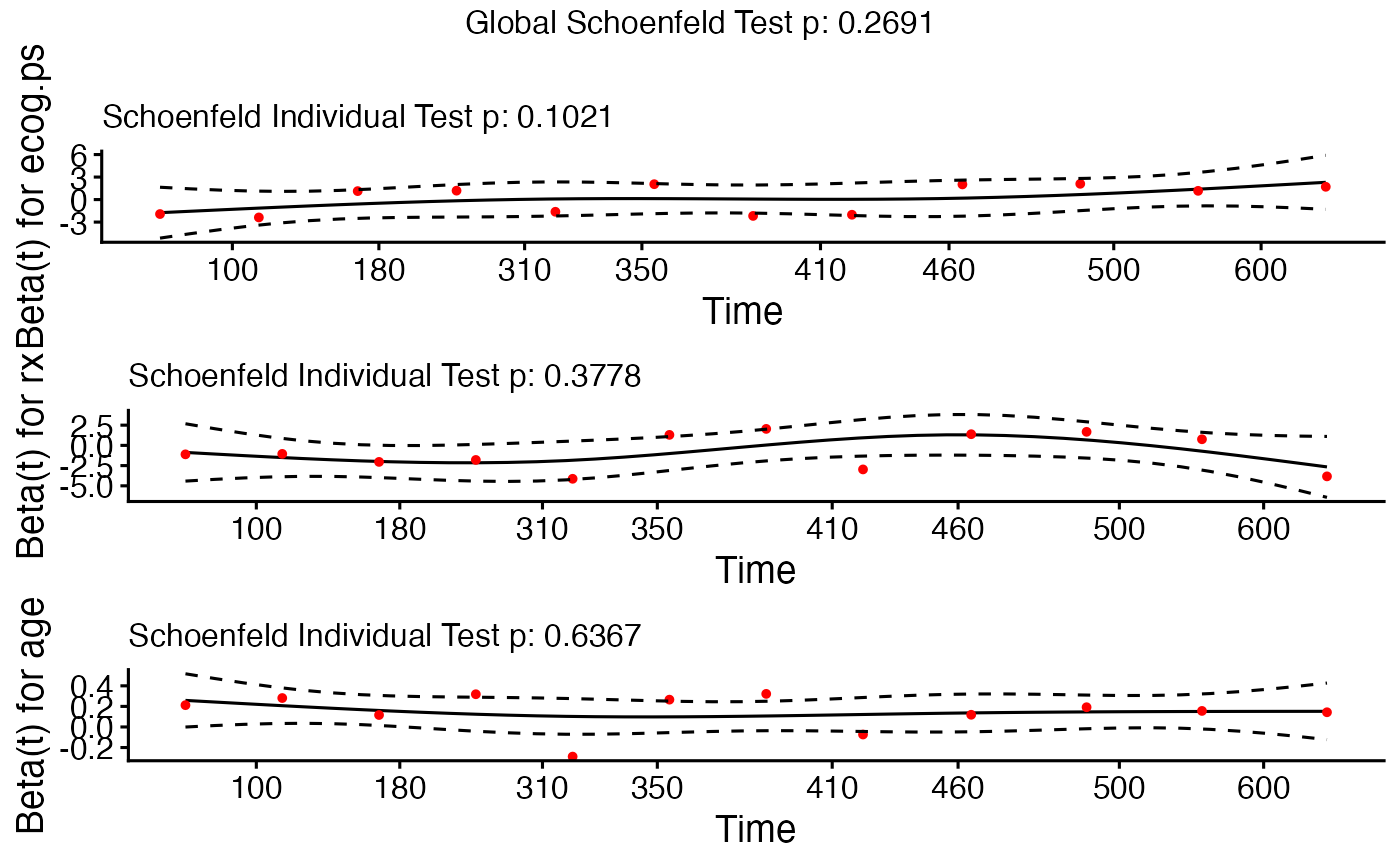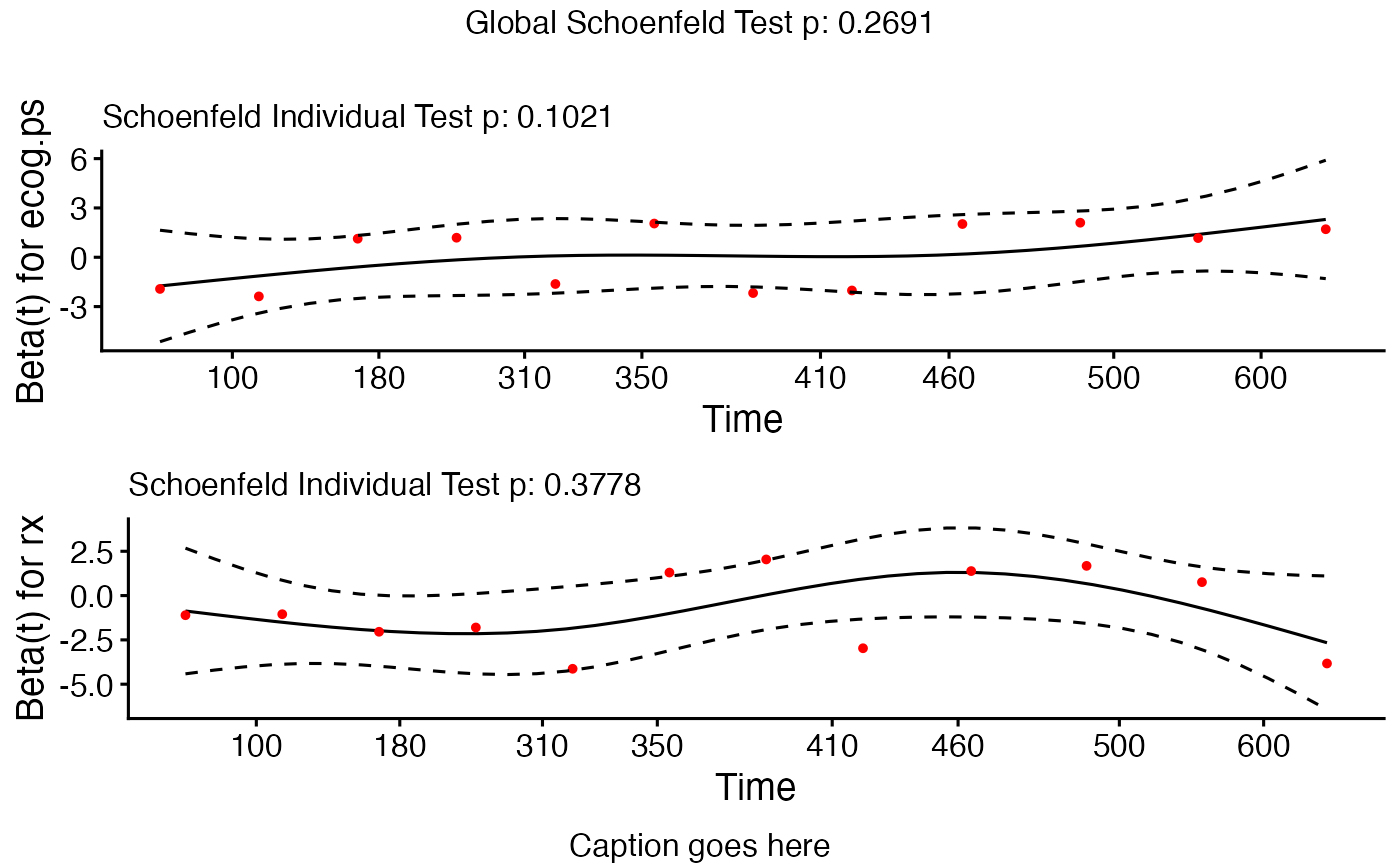Displays a graph of the scaled Schoenfeld residuals, along with a smooth curve using ggplot2. Wrapper around plot.cox.zph.

ggcoxzph(
fit,
resid = TRUE,
se = TRUE,
df = 4,
nsmo = 40,
var,
point.col = "red",
point.size = 1,
point.shape = 19,
point.alpha = 1,
caption = NULL,
ggtheme = theme_survminer(),
...
)

# S3 method for ggcoxzph
print(x, ..., newpage = TRUE)

## Arguments

fit an object of class cox.zph. a logical value, if TRUE the residuals are included on the plot, as well as the smooth fit. a logical value, if TRUE, confidence bands at two standard errors will be added. the degrees of freedom for the fitted natural spline, df=2 leads to a linear fit. number of points used to plot the fitted spline. the set of variables for which plots are desired. By default, plots are produced in turn for each variable of a model. color, size, shape and visibility to be used for points. the caption of the final grob (bottom in arrangeGrob) function, ggplot2 theme name. Allowed values include ggplot2 official themes: see theme. further arguments passed to either the print() function or to the ggpar function for customizing the plot (see Details section). an object of class ggcoxzph open a new page. See grid.arrange.

## Value

Returns an object of class ggcoxzph which is a list of ggplots.

## Details

Customizing the plots: The plot can be easily customized using additional arguments to be passed to the function ggpar(). Read ?ggpubr::ggpar. These arguments include font.main,font.submain,font.caption,font.x,font.y,font.tickslab,font.legend: a vector of length 3 indicating respectively the size (e.g.: 14), the style (e.g.: "plain", "bold", "italic", "bold.italic") and the color (e.g.: "red") of main title, subtitle, caption, xlab and ylab and axis tick labels, respectively. For example font.x = c(14, "bold", "red"). Use font.x = 14, to change only font size; or use font.x = "bold", to change only font face.

## Functions

• ggcoxzph: Graphical Test of Proportional Hazards using ggplot2.

## Examples


library(survival)
fit <- coxph(Surv(futime, fustat) ~ age + ecog.ps + rx, data=ovarian)
cox.zph.fit <- cox.zph(fit)
# plot all variables
ggcoxzph(cox.zph.fit)# plot all variables in specified order
ggcoxzph(cox.zph.fit, var = c("ecog.ps", "rx", "age"), font.main = 12)# plot specified variables in specified order
ggcoxzph(cox.zph.fit, var = c("ecog.ps", "rx"), font.main = 12, caption = "Caption goes here")